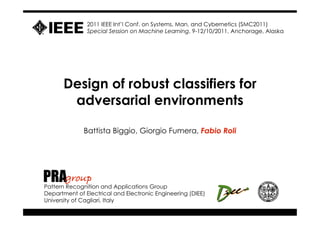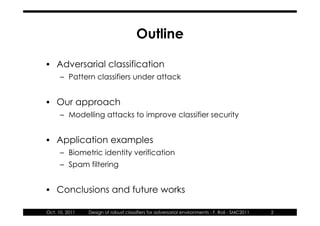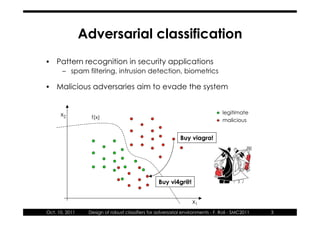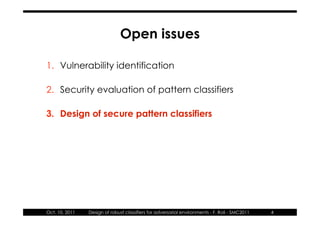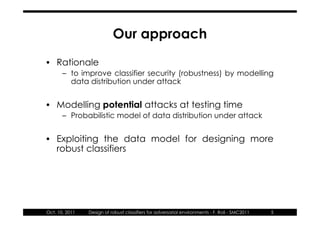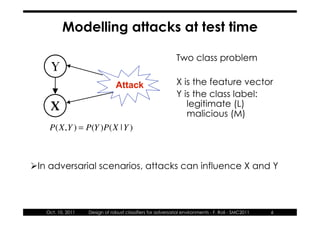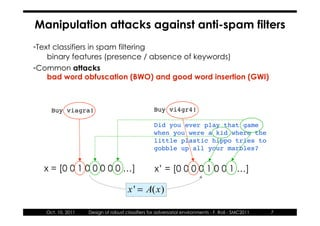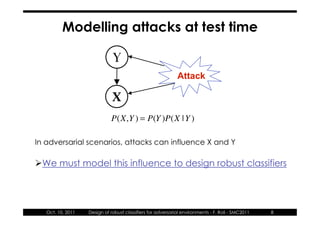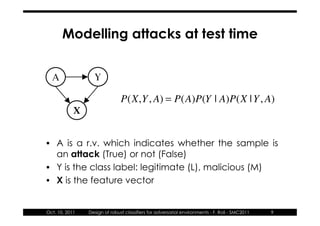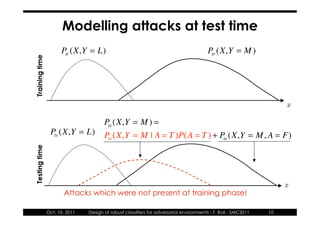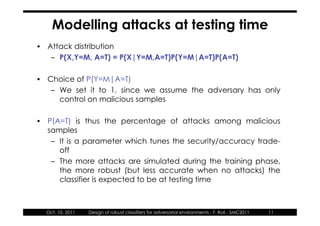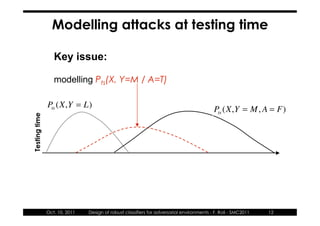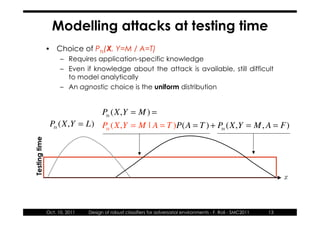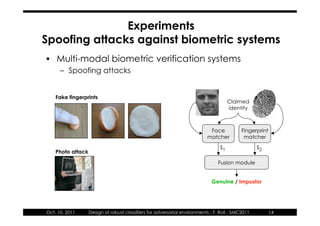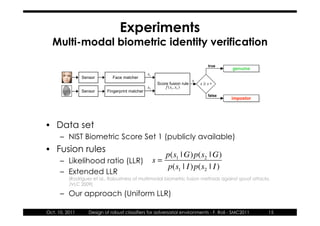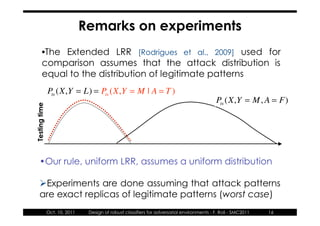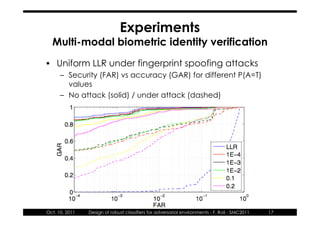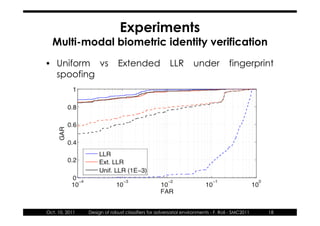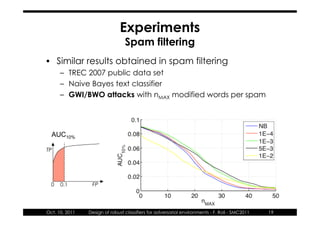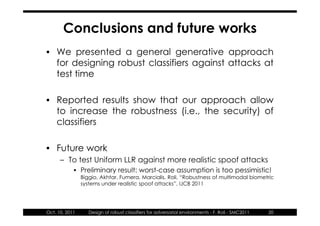1 of 20

### Design of robust classifiers for adversarial environments - Systems, Man, and Cybernetics (SMC), 2011 IEEE International Conference on

1. 2011 IEEE Int’l Conf. on Systems, Man, and Cybernetics (SMC2011) Special Session on Machine Learning, 9-12/10/2011, Anchorage, Alaska Design of robust classifiers for adversarial environments Battista Biggio, Giorgio Fumera, Fabio Roli PRAgroup Pattern Recognition and Applications Group Department of Electrical and Electronic Engineering (DIEE) University of Cagliari, Italy
2. Outline • Adversarial classification – Pattern classifiers under attack • Our approach – Modelling attacks to improve classifier security • Application examples – Biometric identity verification – Spam filtering • Conclusions and future works Oct. 10, 2011 Design of robust classifiers for adversarial environments - F. Roli - SMC2011 2
3. Adversarial classification • Pattern recognition in security applications – spam filtering, intrusion detection, biometrics • Malicious adversaries aim to evade the system x2 legitimate f(x) malicious Buy viagra! Buy vi4gr@! x1 Oct. 10, 2011 Design of robust classifiers for adversarial environments - F. Roli - SMC2011 3
4. Open issues 1. Vulnerability identification 2. Security evaluation of pattern classifiers 3. Design of secure pattern classifiers Oct. 10, 2011 Design of robust classifiers for adversarial environments - F. Roli - SMC2011 4
5. Our approach • Rationale – to improve classifier security (robustness) by modelling data distribution under attack • Modelling potential attacks at testing time – Probabilistic model of data distribution under attack • Exploiting the data model for designing more robust classifiers Oct. 10, 2011 Design of robust classifiers for adversarial environments - F. Roli - SMC2011 5
6. Modelling attacks at test time Two class problem Y Attack X is the feature vector Y is the class label: X legitimate (L) malicious (M) P(X,Y ) = P(Y )P(X | Y ) In adversarial scenarios, attacks can influence X and Y Oct. 10, 2011 Design of robust classifiers for adversarial environments - F. Roli - SMC2011 6
7. Manipulation attacks against anti-spam filters •Text classifiers in spam filtering binary features (presence / absence of keywords) •Common attacks bad word obfuscation (BWO) and good word insertion (GWI) Buy viagra! Buy vi4gr4! Did you ever play that game when you were a kid where the little plastic hippo tries to gobble up all your marbles? x = [0 0 1 0 0 0 0 0 …] x’ = [0 0 0 0 1 0 0 1 …] x ' = A(x) Oct. 10, 2011 Design of robust classifiers for adversarial environments - F. Roli - SMC2011 7
8. Modelling attacks at test time Y Attack X P(X,Y ) = P(Y )P(X | Y ) In adversarial scenarios, attacks can influence X and Y We must model this influence to design robust classifiers Oct. 10, 2011 Design of robust classifiers for adversarial environments - F. Roli - SMC2011 8
9. Modelling attacks at test time A Y P(X,Y , A) = P(A)P(Y | A)P(X | Y , A) X • A is a r.v. which indicates whether the sample is an attack (True) or not (False) • Y is the class label: legitimate (L), malicious (M) • X is the feature vector Oct. 10, 2011 Design of robust classifiers for adversarial environments - F. Roli - SMC2011 9
10. Modelling attacks at test time Ptr (X,Y = L) Ptr (X,Y = M ) Training time x Pts (X,Y = M ) = Pts (X,Y = L) Pts (X,Y = M | A = T )P(A = T ) + Pts (X,Y = M , A = F) Testing time x Attacks which were not present at training phase! Oct. 10, 2011 Design of robust classifiers for adversarial environments - F. Roli - SMC2011 10
11. Modelling attacks at testing time • Attack distribution – P(X,Y=M, A=T) = P(X|Y=M,A=T)P(Y=M|A=T)P(A=T) • Choice of P(Y=M|A=T) – We set it to 1, since we assume the adversary has only control on malicious samples • P(A=T) is thus the percentage of attacks among malicious samples – It is a parameter which tunes the security/accuracy trade- off – The more attacks are simulated during the training phase, the more robust (but less accurate when no attacks) the classifier is expected to be at testing time Oct. 10, 2011 Design of robust classifiers for adversarial environments - F. Roli - SMC2011 11
12. Modelling attacks at testing time Key issue: modelling Pts(X, Y=M / A=T) Pts (X,Y = L) Pts (X,Y = M , A = F) Testing time Oct. 10, 2011 Design of robust classifiers for adversarial environments - F. Roli - SMC2011 12
13. Modelling attacks at testing time • Choice of Pts(X, Y=M / A=T) – Requires application-specific knowledge – Even if knowledge about the attack is available, still difficult to model analytically – An agnostic choice is the uniform distribution Pts (X,Y = M ) = Pts (X,Y = L) Pts (X,Y = M | A = T )P(A = T ) + Pts (X,Y = M , A = F) Testing time x Oct. 10, 2011 Design of robust classifiers for adversarial environments - F. Roli - SMC2011 13
14. Experiments Spoofing attacks against biometric systems • Multi-modal biometric verification systems – Spoofing attacks Fake fingerprints Claimed identity Face Fingerprint matcher matcher s1 s2 Photo attack Fusion module Genuine / Impostor Oct. 10, 2011 Design of robust classifiers for adversarial environments - F. Roli - SMC2011 14
15. Experiments Multi-modal biometric identity verification true genuine s1 Sensor Face matcher s Score fusion rule s ! s" s2 f (s1 , s2 ) Sensor Fingerprint matcher false impostor • Data set – NIST Biometric Score Set 1 (publicly available) • Fusion rules p(s1 | G)p(s2 | G) – Likelihood ratio (LLR) s= p(s1 | I )p(s2 | I ) – Extended LLR [Rodrigues et al., Robustness of multimodal biometric fusion methods against spoof attacks, JVLC 2009] – Our approach (Uniform LLR) Oct. 10, 2011 Design of robust classifiers for adversarial environments - F. Roli - SMC2011 15
16. Remarks on experiments •The Extended LRR [Rodrigues et al., 2009] used for comparison assumes that the attack distribution is equal to the distribution of legitimate patterns Pts (X,Y = L) = Pts (X,Y = M | A = T ) Pts (X,Y = M , A = F) Testing time •Our rule, uniform LRR, assumes a uniform distribution Experiments are done assuming that attack patterns are exact replicas of legitimate patterns (worst case) Oct. 10, 2011 Design of robust classifiers for adversarial environments - F. Roli - SMC2011 16
17. Experiments Multi-modal biometric identity verification • Uniform LLR under fingerprint spoofing attacks – Security (FAR) vs accuracy (GAR) for different P(A=T) values – No attack (solid) / under attack (dashed) Oct. 10, 2011 Design of robust classifiers for adversarial environments - F. Roli - SMC2011 17
18. Experiments Multi-modal biometric identity verification • Uniform vs Extended LLR under fingerprint spoofing Oct. 10, 2011 Design of robust classifiers for adversarial environments - F. Roli - SMC2011 18
19. Experiments Spam filtering • Similar results obtained in spam filtering – TREC 2007 public data set – Naive Bayes text classifier – GWI/BWO attacks with nMAX modified words per spam AUC10% TP 0 0.1 FP Oct. 10, 2011 Design of robust classifiers for adversarial environments - F. Roli - SMC2011 19
20. Conclusions and future works • We presented a general generative approach for designing robust classifiers against attacks at test time • Reported results show that our approach allow to increase the robustness (i.e., the security) of classifiers • Future work – To test Uniform LLR against more realistic spoof attacks • Preliminary result: worst-case assumption is too pessimistic! Biggio, Akhtar, Fumera, Marcialis, Roli, “Robustness of multimodal biometric systems under realistic spoof attacks”, IJCB 2011 Oct. 10, 2011 Design of robust classifiers for adversarial environments - F. Roli - SMC2011 20